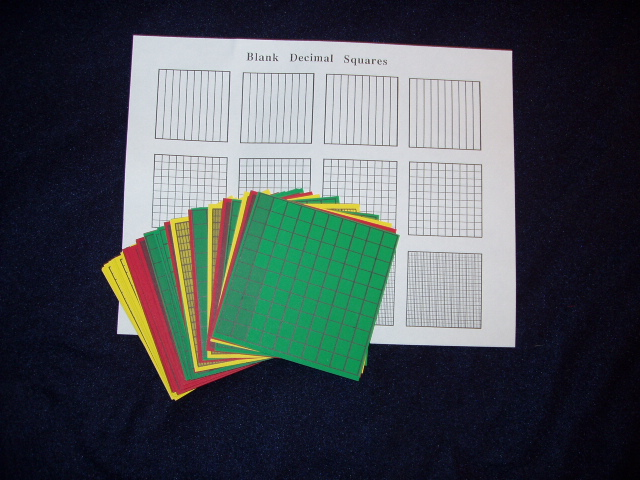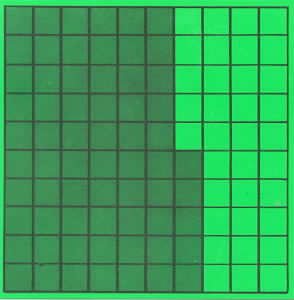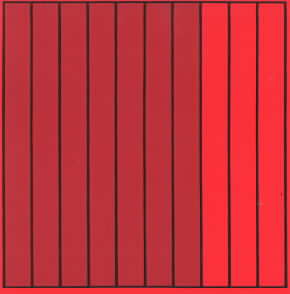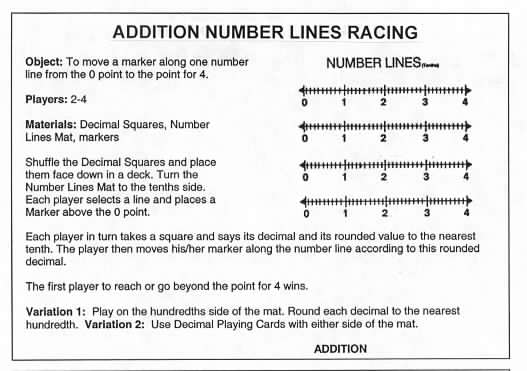Home
 Addition and Subtraction .Materials: Deck of Decimal Squares per group and sheets of Blank Decimal Squares for Tenths Hundredths and Thousandths . Addition (combining the shaded parts of two squares) and subtraction (comparing the difference in shaded amounts of two squares) is modeled in many of the games and activities in the Decimal Squares Teacher's Guide (see Contents and List of Games). Such activities help to show that the basic ideas of addition and subtraction are the same for decimals as for whole numbers that is the concepts do not change when we go from whole numbers to decimals. Some of these activities are shown here and can be carried out by using verbal descriptions of the squares (see part c) or by recording the results with decimals.: . (a) Select an arbitrary square describe its shaded amount and then describe how many more shaded parts are needed to make one whole square. (b) Select two squares and determine the total shaded amount. Ask for a show of hands for people with: less than one whole square; more than one whole square; one whole square. c.) Select two squares of different colors and then determine: the total shaded amount; and the difference in the shaded amounts of the two squares. Ask for one or two volunteers to describe their squares and the sums and differences both without and with decimals. Examples of descriptions are shown here.Sum: The total shaded amount of a square with 65 parts out of 100 and a square with 7 parts out of 10 is one whole square and 35 parts out of 100. Or + .7 = 1.35. Difference: The difference in shaded amounts of a square with 7 parts out of 10 and a square with 65 parts out of 100 is a square with 5 parts out of 100. Or - .65 = .05. These students are playing Concentration by trying to find two squares whose total shaded amount is one whole square. It looks like they might be playing cooperatively or in teams.Photo courtesy of Herb Moyer NUMBER LINES RACING There are two versions of the following game one for addition (see rules below) and one for subtraction. These games are on pages 83 and 87 of the Decimal Squares Teacher’s Guide and involve adding amounts on a number line and mixed numbers. Show a transparency of the Number Lines from the end pages of the Decimal Squares Teacher’s Guide and briefly illustrate one or two moves in the game. .Activity - Shade Blank Decimal Squares to illustrate the following operations: .7 + .6; .43 + .92; .84 - .37; and .65 - .4.